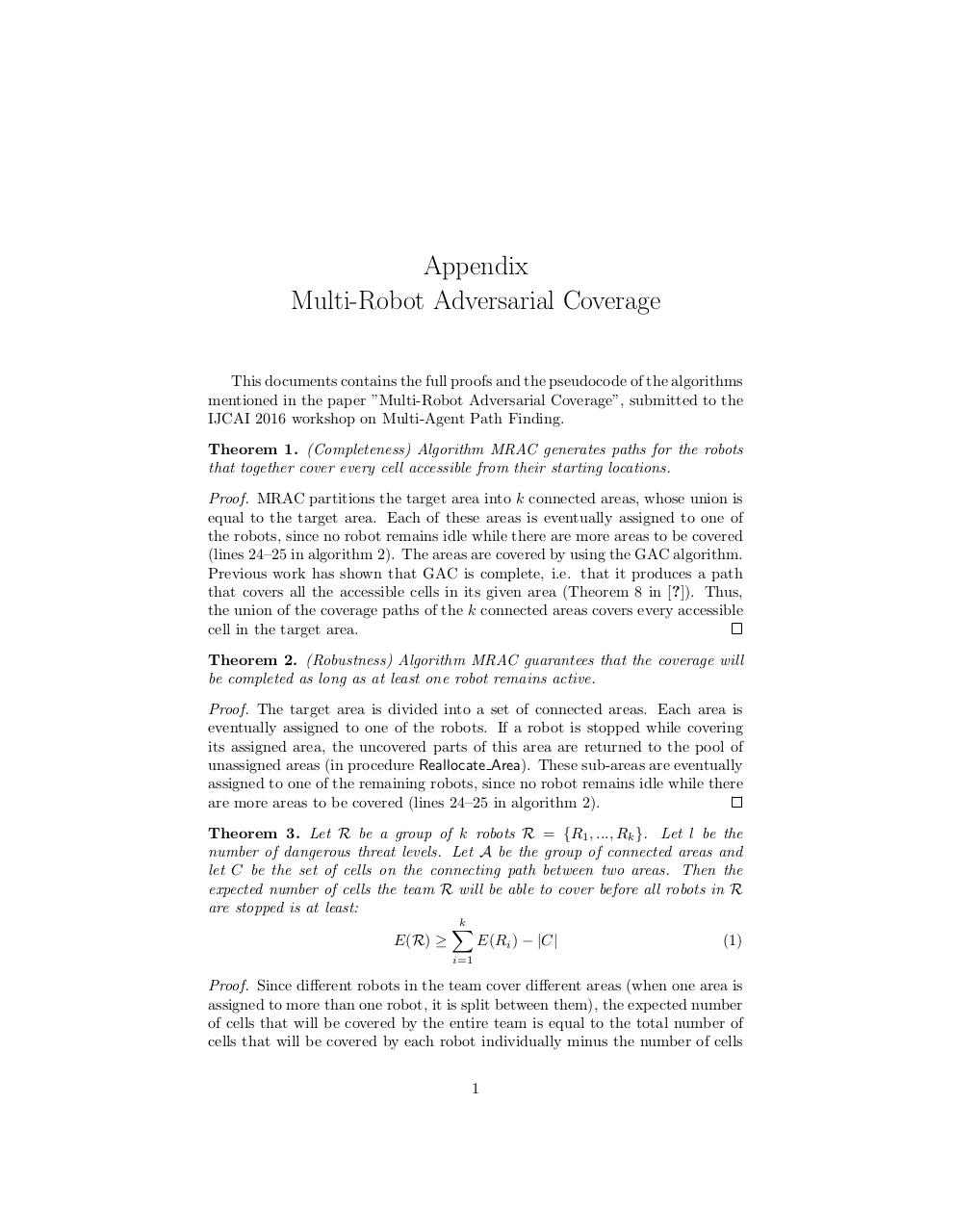Page 1 2 3 4 5 6 7 8

#### Text preview

Appendix
This documents contains the full proofs and the pseudocode of the algorithms
mentioned in the paper ”Multi-Robot Adversarial Coverage”, submitted to the
IJCAI 2016 workshop on Multi-Agent Path Finding.
Theorem 1. (Completeness) Algorithm MRAC generates paths for the robots
that together cover every cell accessible from their starting locations.
Proof. MRAC partitions the target area into k connected areas, whose union is
equal to the target area. Each of these areas is eventually assigned to one of
the robots, since no robot remains idle while there are more areas to be covered
(lines 24–25 in algorithm 2). The areas are covered by using the GAC algorithm.
Previous work has shown that GAC is complete, i.e. that it produces a path
that covers all the accessible cells in its given area (Theorem 8 in [?]). Thus,
the union of the coverage paths of the k connected areas covers every accessible
cell in the target area.
Theorem 2. (Robustness) Algorithm MRAC guarantees that the coverage will
be completed as long as at least one robot remains active.
Proof. The target area is divided into a set of connected areas. Each area is
eventually assigned to one of the robots. If a robot is stopped while covering
its assigned area, the uncovered parts of this area are returned to the pool of
unassigned areas (in procedure Reallocate Area). These sub-areas are eventually
assigned to one of the remaining robots, since no robot remains idle while there
are more areas to be covered (lines 24–25 in algorithm 2).
Theorem 3. Let R be a group of k robots R = {R1 , ..., Rk }. Let l be the
number of dangerous threat levels. Let A be the group of connected areas and
let C be the set of cells on the connecting path between two areas. Then the
expected number of cells the team R will be able to cover before all robots in R
are stopped is at least:
k
X
E(R) ≥
E(Ri ) − |C|
(1)
i=1

Proof. Since different robots in the team cover different areas (when one area is
assigned to more than one robot, it is split between them), the expected number
of cells that will be covered by the entire team is equal to the total number of
cells that will be covered by each robot individually minus the number of cells
1